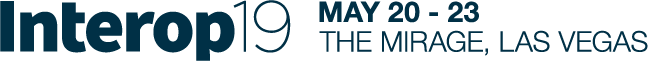Commentary

# Anomaly Detection Techniques: Defining Normal

The challenge is identifying suspicious events in training sets where no anomalies are encountered. Part two of a two-part series.

The problem of anomaly detection is not new, and a number of solutions have already been proposed over the years. However, before starting with the list of techniques, let's agree on a necessary premise: All anomaly detection techniques must involve a training set where no anomaly examples are encountered. The challenge consists of identifying suspicious events, even in the absence of examples.

We talk in this case of a training set formed of only "normal" events. The definition of "normal" is, of course, arbitrary. In the case of anomaly detection, a "normal" event refers just to the events represented in the training set. Here are four common approaches.

Statistical Methods
Everything that falls outside of the statistical distribution calculated over the training set is considered an anomaly.

The simplest statistical method is the control chart. Here the average and standard deviation for each feature is calculated on the training set. Thresholds are then defined around the average value as k*std deviation where k is an arbitrary coefficient, usually between 1.5 and 3.0, depending on how conservative we want the algorithm to be. During deployment, a point trespassing the thresholds in both directions is a suspicious candidate for an anomaly event.

Such methods are easy to implement and understand, fast to execute, and fit both static and time series data. However, they might be too simple to detect more subtle anomalies.

Clustering
Other proposed methods are often clustering methods. Since the anomaly class is missing from the training set, clustering algorithms might sound suitable for the task.

The concept here is clear. The algorithm creates a number of clusters on the training set. During deployment, the distance between the current data point and the clusters is calculated. If the distance is above a given threshold, the data point becomes a suspicious candidate for an anomaly event. Depending on the distance measure used and on the aggregation rules, different clustering algorithms have been designed and different clusters are created.

This approach, however, does not fit time series data since a fixed set of clusters cannot capture the evolution in time.

Supervised Machine Learning
Surprised? Supervised machine learning algorithms can also be used for anomaly detection. They would even cover all data situations since supervised machine learning techniques can be applied to static classification as well as to time series prediction problems. However, since they all require a set of examples for all involved classes, we need a little change in perspective.

In the case of anomaly detection, a supervised machine learning model can only be trained on "normal" data — i.e., on data describing the system operating in "normal" conditions. The evaluation of whether the input data is an anomaly can only happen during deployment after the classification/prediction has been made.There are two popular approaches for anomaly detection relying on supervised learning techniques.

The first one is a neural autoassociator (or autoencoder). The autoassociator is trained to reproduce the input pattern onto the output layer. The pattern reproduction works fine as long as the input patterns are similar to the examples in the training set — i.e., “normal.” Things do not work quite as well when a new, different shape vector appears at the input layer. In this case, the network will not be able to adequately reproduce the input vector onto the output layer. If a distance is calculated between the input and the output of the network, the distance value will be higher for an anomaly rather than for a "normal" event. Again, defining a threshold on this distance measure should find the anomaly candidates. This approach works well for static data points but does not fit time series data.

The second approach uses algorithms for time series prediction. The model is trained to predict the value of the next sample based on the history of previous n samples on a training set of "normal" values. During deployment, the prediction of the next sample value will be relatively correct — i.e., close to the real sample value, if the past history comes from a system working in "normal" conditions. The predicted value will be farther from reality if the past history samples come from a system not working in "normal" conditions anymore. In this case, a distance measure calculated between the predicted sample value and the real sample value would isolate candidates for anomaly events.

Related Content:Join Dark Reading LIVE for two cybersecurity summits at Interop 2019. Learn from the industry's most knowledgeable IT security experts. Check out the Interop agenda here.